## Poisson Trials

A numberTrials in which the probability of successvaries from trial to trial. Letbe the number of successes, then(1)

whereis the Variance ofand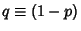. Uspensky has shown that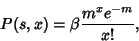(2)

where(3)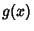(4)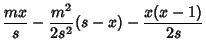(5)

and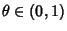. The probability that the number of successes is at leastis given by(6)

Uspensky gives the true probability that there are at leastsuccesses intrials as(7)

where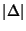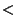(8)(9)